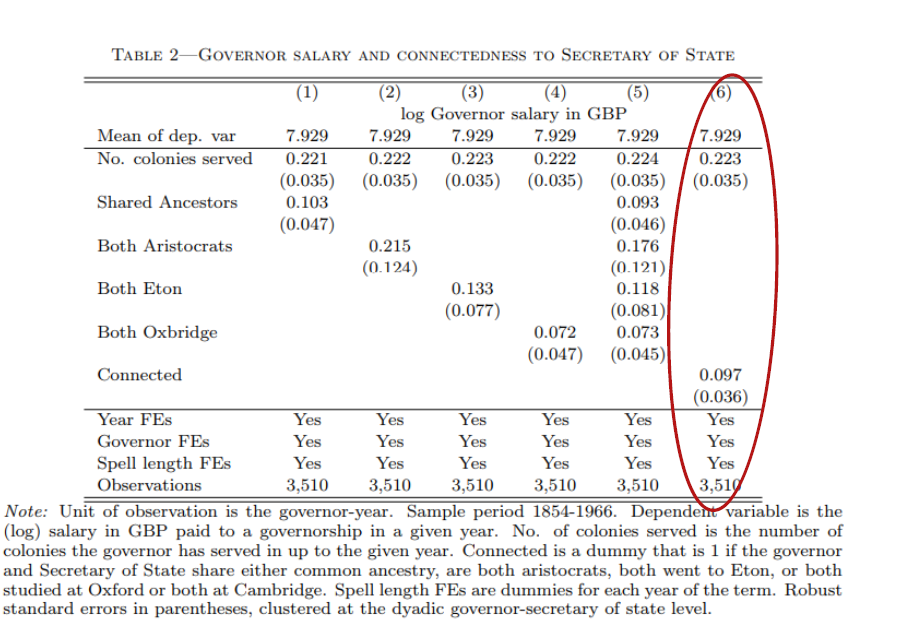# reghdfe：多维面板固定效应估计

Stata 连享会   主页 || 视频 || 推文

reghdfe：多维面板固定效应估计

## 1. 命令的安装

``````ssc install gtools, replace
ssc install reghdfe, replace // 安装最新版命令
``````

``````. which ftools
*! version 2.37.0 16aug2019

. which reghdfe
*! version 5.7.3 13nov2019
``````

## 2. 命令的语法

``````reghdfe depvar [indepvars] [if] [in] [weight], absorb(absvars) [options]
``````

• `depvar`: 因变量
• `indepvars`: 解释变量
• `absorb(absvars)`：引入固定效应
• 可以包含多维固定效应，即 `absorb (var1,var2,var3,...)`。若想保存对某变量的固定效应，则运行命令`absorb (var1,var2,FE3=var3)` ， 变量 FE3 将保存对 var3 的固定效应估计结果。
• 可以包含不同效应间的交互影响，即`absorb(var1#var2)`

## 3. 命令的操作

### 3.1 估计双重差分的固定效应模型（DID)

``````. set obs 400
. gen firm=_n  ///生成企业数量
. expand 24
. bysort firm: gen t=_n  ///时间跨度设定为24个季度（6年）
. gen d=(t>=14)
. label var d "=1 if post-treatment" ///设定事件冲击发生在第14期
. gen r=rnormal()
. qui sum r, d
. bysort firm: gen i=(r>=r(p50)) if _n==1
. bysort firm: replace i=i[_n-1] if i==. & _n!=1 ///设定处理组和对照组
. drop r
. label var i "=1 if treated group, =0 if untreated group"
. gen e = rnormal() ///设定随机变量
. label var e "normal random variable"
. gen y = 0.3 + 0.19*i + 1.67*d + 0.56*i*d + e ///模型设置
``````

``````gen did = i*d ///生成交互项
reghdfe y did, absorb(firm t) vce(cluster firm)
``````

``````. reghdfe y did, absorb(firm t) vce(cluster firm)
(MWFE estimator converged in 2 iterations)

HDFE Linear regression                            Number of obs   =      9,600
Absorbing 2 HDFE groups                           F(   1,    399) =     175.80
Statistics robust to heteroskedasticity           Prob > F        =     0.0000
R-squared       =     0.5102
Within R-sq.    =     0.0198
Number of clusters (firm)    =        400         Root MSE        =     1.0043

(Std. Err. adjusted for 400 clusters in firm)
------------------------------------------------------------------------------
|               Robust
y |      Coef.   Std. Err.      t    P>|t|     [95% Conf. Interval]
-------------+----------------------------------------------------------------
did |   .5656247   .0426601    13.26   0.000     .4817581    .6494914
_cons |   1.143579   .0084565   135.23   0.000     1.126954    1.160204
------------------------------------------------------------------------------

Absorbed degrees of freedom:
-----------------------------------------------------+

Absorbed FE | Categories  - Redundant  = Num. Coefs |
-------------+---------------------------------------|
firm |       400         400           0    *|
t |        24           0          24     |
-----------------------------------------------------+
* = FE nested within cluster; treated as redundant for DoF computation

``````

### 3.2 估计多维固定效应的线性模型（复制一篇 AER 论文）Source: Xu, G. (2018). The Costs of Patronage: Evidence from the British Empire. American Economic Review, 108 (11): 3170-98.

• $log{w}_{ist}$为政府官员 $i$ 于时间 $t$ 在 $j$ 州执政时的对数工资水平。Stata 命令中，该变量名为 log_salary_governor_gbp
• ${c}_{it}$ 为虚拟变量（Dummy Variable)，当政府官员与其上任官员存在社会联系时，该变量取1。如若不然，则取0。社会联系包括：共同祖先，贵族身份以及教育背景。Stata 命令中，该变量名为 connected
• ${\theta }_{i}$ 为政府官员固定效应。该部分的设置为了解决政府官员的异质性 (heterogeneity) 问题。例如，具有较强能力的政府官员更有可能建立更多的社会关系。Stata 命令中, aid 为不同官员的 unique ID 变量。
• ${\omega }_{i}$ 为政府官员执政时长固定效应。设置该部分是因为，执政时间的长短可能也会对社会关系产生影响。Stata 命令中, duration 为官员执政时长变量。
• ${x}_{it}^{\prime }$ 为控制变量。作者选用了执政者在历史上执政过的州的数目。Stata 命令中，该变量名为 no_colonies
• ${\gamma }_{t}$ 为年份固定效应。该部分的设置是为了吸收执政者们在不同时期受到的共同时间冲击。Stata 命令中, year 为年份变量。
• ${ϵ}_{ist}$ 为残差。作者使用了聚类标准误的方法。

``````reghdfe log_salary_governor_gbp no_colonies connected, ///
absorb(aid year duration) vce(cluster bilateral)
``````

``````. quietly use "analysis.dta", replace
. reghdfe log_salary_governor_gbp no_colonies connected, ///
absorb(aid year duration) vce(cluster bilateral)
(MWFE estimator converged in 26 iterations)

HDFE Linear regression                            Number of obs   =      3,510
Absorbing 3 HDFE groups                           F(   2,   1517) =      25.45
Statistics robust to heteroskedasticity           Prob > F        =     0.0000
R-squared       =     0.9255
Within R-sq.    =     0.0978
Number of clusters (bilateral) =      1,518       Root MSE        =     0.2374

(Std. Err. adjusted for 1,518 clusters in bilateral)
------------------------------------------------------------------------------
|               Robust
log_salary~p |      Coef.   Std. Err.      t    P>|t|     [95% Conf. Interval]
-------------+----------------------------------------------------------------
no_colonies |   .2234767   .0347473     6.43   0.000     .1553189    .2916346
connected |   .0972969   .0355508     2.74   0.006     .0275628    .1670309
_cons |   7.485619    .065766   113.82   0.000     7.356617    7.614621
------------------------------------------------------------------------------

Absorbed degrees of freedom:
-----------------------------------------------------+
Absorbed FE | Categories  - Redundant  = Num. Coefs |
-------------+---------------------------------------|
aid |       456           0         456     |
year |       110           1         109     |
duration |         7           1           6    ?|
-----------------------------------------------------+
? = number of redundant parameters may be higher

``````

## 文献来源

• Correia, S. (2016). Linear Models with High-Dimensional Fixed Effects: An Efficient and Feasible Estimator, Working Paper. [PDF]
• Duflo, E. (2004). The medium run effects of educational expansion: Evidence from a large school construction program in Indonesia. Journal of Development Economics, 74(1), 163-197. [PDF]
• Xu, G. (2018). The Costs of Patronage: Evidence from the British Empire. American Economic Review, 108 (11): 3170-98. [PDF]

## 相关课程

http://lianxh.duanshu.com

### 课程一览

Stata数据清洗 游万海 直播, 2 小时，已上线

Note: 部分课程的资料，PPT 等可以前往 连享会-直播课 主页查看，下载。

#### 关于我们

• Stata连享会 由中山大学连玉君老师团队创办，定期分享实证分析经验。直播间 有很多视频课程，可以随时观看。
• 连享会-主页知乎专栏，300+ 推文，实证分析不再抓狂。
• 公众号推文分类： 计量专题 | 分类推文 | 资源工具。推文分成 内生性 | 空间计量 | 时序面板 | 结果输出 | 交乘调节 五类，主流方法介绍一目了然：DID, RDD, IV, GMM, FE, Probit 等。
• 公众号关键词搜索/回复 功能已经上线。大家可以在公众号左下角点击键盘图标，输入简要关键词，以便快速呈现历史推文，获取工具软件和数据下载。常见关键词：`课程, 直播, 视频, 客服, 模型设定, 研究设计, stata, plus, 绘图, 编程, 面板, 论文重现, 可视化, RDD, DID, PSM, 合成控制法`